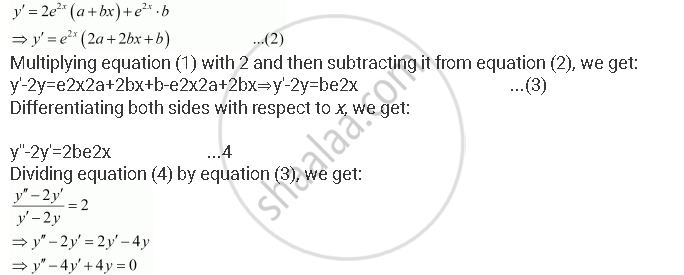Share

# Form a Differential Equation Representing the Given Family of Curves by Eliminating Arbitrary Constants a and B. Y = E2x (A + Bx) - CBSE (Commerce) Class 12 - Mathematics

ConceptFormation of a Differential Equation Whose General Solution is Given

#### Question

Form a differential equation representing the given family of curves by eliminating arbitrary constants a and b.

y = e2x (a + bx)

#### Solution

y = e2x (a + bx) ...(1)

Differentiating both sides with respect to x, we get:This is the required differential equation of the given curve.

Is there an error in this question or solution?

#### APPEARS IN

NCERT Solution for Mathematics Textbook for Class 12 (2018 to Current)
Chapter 9: Differential Equations
Q: 4 | Page no. 391

#### Video TutorialsVIEW ALL 

Solution Form a Differential Equation Representing the Given Family of Curves by Eliminating Arbitrary Constants a and B. Y = E2x (A + Bx) Concept: Formation of a Differential Equation Whose General Solution is Given.
S